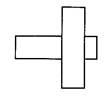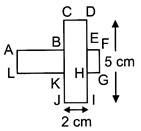Courses

# The given figure is obtained by placing a rectangle on top of another identical rectangle. The length of a rectangle is 5 cm and its breadth is 2 cm. Find the perimeter of the figure.a)20 cm                      b)15 cm              c)14 cm                 d)18 cmCorrect answer is option 'A'. Can you explain this answer? Related Test: Test: Building With Bricks - 5

## Class 4 Question

By Rajesh Singh · Apr 17, 2020 ·Class 4
KUNCHANAPALLI MANOHAR answered Apr 18, 2020
Perimeter of the figure = AB + BC + CD + DE + EF + FG + GH + HI + IJ + JK + KL + LA= (AB + CD + FE) + (BC + AL + JK) + (DE + FG + HI) + (GH +IJ + KL)